# The Knitro MPS file reader

Knitro implements a MPS file reader to import optimization problems specified in MPS and extended MPS formats. Knitro’s parser uses by default the free format MPS-style, but supports as well the fixed MPS format.

Note that MPS files should be encoded as ASCII files.

To ensure that Knitro parses correctly the MPS file, the names of rows and columns should not have any blank spaces.

```CORRECT:
name_variable
UNCORRECT:
name variable
```

The maximal length of a variable’s name is set by default at 512 (the user can set the `KN_MPS_LENGTH_NAME` option to change this setting).

## Free MPS format

### Example

An example of a MPS file is given below:

```NAME          TESTPROB
ROWS
N  COST
L  LIM1
G  LIM2
E  MYEQN
COLUMNS
XONE      COST                 1   LIM1                 1
XONE      LIM2                 1
YTWO      COST                 4   LIM1                 1
YTWO      MYEQN               -1
ZTHREE    COST                 9   LIM2                 1
ZTHREE    MYEQN                1
RHS
RHS1      LIM1                 5   LIM2                10
RHS1      MYEQN                7
BOUNDS
UP BND1      XONE                 4
LO BND1      YTWO                -1
UP BND1      YTWO                 1
ENDATA
```

We discuss hereafter the different flags used in the (extended) free MPS format.

In the sequel, `_` indicates a blank space.

### NAME

Name of the problem. This section contains at most one line.

### OBJSENSE (optional)

Sense of the objective function. This section contains at most one line with shape

```SENSE
```

`SENSE` specifies the objective’s sense, with value being either

```MAX
MIN
```

By default, the sense is set at `MIN`.

### OBJNAME (optional)

Name of the objective. This section contains at most one line. By default, no name is set.

### ROWS

In this section, each line specifies a row of the problem with shape

```_S   rowname
```

The `S` character specifies the sense of the current row, with possible values being

```E   equality constraint
G   greater than equal
L   less than equal
N   non specified
```

The objective line is specified by a `N` flag. If more than one line has a `N` flag, the objective is assumed to be the first `N` flag encountered.

### COLUMNS

Each line in this section specifies a column of the problem with shape

```_cname1  rname1  value1  [rname2]    [value2]
```

With

```cname1    name of the variable corresponding to current row
rname1    name of a constraint where the row appears
value1    numerical values corresponding to row's coefficient in constraint
[rname2]  name of a second constraint where the row appears
[value2]  numerical values corresponding to row's coefficient in second constraint
```

Elements inside brackets `[]` are optional. Note that `rname1` and `rname2` should be present in the `ROWS` section.

Columns’ names should be consecutive. Otherwise, the MPS file could not be parsed correctly.

```CORRECT:
_cname1
_cname1
_cname2

UNCORRECT:
_cname1
_cname2
_cname1
```

### RHS (optional)

This section specifies the right-hand side of the different constraints. Each line is shaped

```_name    cname1  value1  [cname2]  [value2]
```

with

```name      name of the RHS vector
cname1    name of a constraint
value1    right-hand side of this constraint
[cname1]  name of a second constraint
[value1]  right-hand side of this second constraint
```

Elements inside brackets `[]` are optional. `cname1` and `cname2` should be present in the `ROWS` section.

If a constraint `cname` presents in the `ROWS` section does not appear in `RHS`, its right-hand side is set at 0 by default.

### BOUNDS (optional)

This section specifies the upper and lower bounds for the variables defined in the `COLUMNS` section. By default, if no bound is specified, the lower bound `LB` is set equal to `0` and the upper bound `UB` is set equal to `KN_INFINITY`.

Each line has the form

```_BO    vname    value
```

with `vname` the name of the variable, `BO` the nature of the bounds, defined as

```FR      Free variable
FX      Fixed variable
LO      Lower bound
UP      Upper bound
MI      Minus infinity
PL      Plus infinity
```

If the same variable appears several times, additional values would be discarded.

Additional values exist to specify integer/binary variables: .. code-block:: none

FR Free variable BV Binary variable UI Upper-bounded integer variable LI Lower-bounded integer variable

### RANGES (optional)

This section specifies constraints that lie inside an interval between two values.

A line inside the `RANGES` section has shape:

```_T  row1    value1    [row2]    [value2]
```

with `[row2]` and `[value2]` optional values. `T` is the right-hand side specifier.

We have the following possibilities used in the `RHS` section.

Row type

Sign

RHS lower limit

RHS upper limit

G

+/-

rhs

rhs + range

L

+/-

rhs - range

rhs

E

+

rhs

rhs + range

E

-

rhs + range

rhs

For instance, the constraint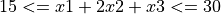is encoded as

```COLUMNS
L  cons1
ROWS
x1    cons1    1
x2    cons1    2
x3    cons1    1
RHS
rhs   cons1    30
RANGES
rhs   cons1    15
```

A quadratic objective function is specified either by a `QUADOBJ` flag or a `QMATRIX` flag. For each quadratic term, the format is

```col1    col2    values
```

with `col1` the name of the first variable and `col2` the name of the second variable, `values` being the coefficient of the term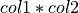in the objective.

The quadratic matrix as an implicit factors of `0.5`. For instance, the objective function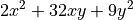is encoded with `QUADOBJ` as

```QUADOBJ
x   x   4
y   y   18
x   y   32
```

and with `QMATRIX` as

```QUADOBJ
x   x   4
y   y   18
x   y   32
y   x   32
```

The term `y * x` is assumed implicit in `QUADOBJ`, thus explaining why the term is not doubled.

The names `x` and `y` should be defined in the `COLUMNS` section.

### QCMATRIX (optional)

Quadratic constraints are specified in `QCMATRIX` sections. Each quadratic constraint `qc_index` is encoded by a dedicated `QCMATRIX` flag:

```QCMATRIX qc_index
some text...
```

Inside a `QCMATRIX` flag, the format is similar as in the `QMATRIX` section, that is, the format of each line is

```col1    col2    values
```

with `col1` the name of the first variable, `col2` the name of the second variable, `values` being the coefficient of the termin the constraint.

The quadratic matrix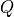defined must be symmetric.

The constraint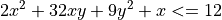is encoded as

```ROWS
L  qc1
COLUMNS
x  qc1  1
y  qc1  0
QCMATRIX qc1
x  x   2
y  y   9
x  y   16
y  x   16
RHS
rhs1  qc1  12
ENDATA
```

For `y` to be defined correctly, it must appear in the `COLUMNS` section even if it does not appear linearly in the `qc1` constraint.

On the contrary of the `QMATRIX` and `QUADOBJ` section, no scaling factor is applied to the definition of the constraint in the solver.

### ENDATA (optional)

Specifies the end of the MPS file.

## C example

In the callable library interface, a MPS file can be loaded through the KN_load_mps_file API function.

```/*---- LOAD MPS FILE ----*/
if (KN_load_mps_file (kc, "file.mps") != 0)
exit( -1 );
```